# Playing with the CPU pipeline

This article will show how basic knowledge of a modern CPU’s instruction pipeline can help micro-optimise code at very little cost, using a real world example: the approximation of a trigonometric function. All this without necessarily having to look at lines of assembly code.

## Evaluating polynomials

Who needs polynomials anyway? We’re writing games, not a computer algebra system, after all. But wait! Taylor series are an excellent mathematical tool for approximating certain classes of functions. For instance, this is the Taylor series of sin(x) near x = 0: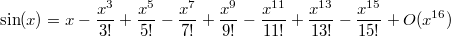Truncating the series at the 15th power will compute sin(x) with an absolute error no greater than 1e-11 in the range [-π/2; π/2], and 2e-16 in the range [-π/4; π/4].

However, a better approximation known as the minimax polynomial (probably featured in an upcoming article) will give a maximum absolute error of about 2e-16 on the whole [-π/2; π/2] range:

static double a0 = +1.0;
static double a1 = -1.666666666666580809419428987894207e-1;
static double a2 = +8.333333333262716094425037738346873e-3;
static double a3 = -1.984126982005911439283646346964929e-4;
static double a4 = +2.755731607338689220657382272783309e-6;
static double a5 = -2.505185130214293595900283001271652e-8;
static double a6 = +1.604729591825977403374012010065495e-10;
static double a7 = -7.364589573262279913270651228486670e-13;
double sin1(double x)
{
return a0 * x
+ a1 * x * x * x
+ a2 * x * x * x * x * x
+ a3 * x * x * x * x * x * x * x
+ a4 * x * x * x * x * x * x * x * x * x
+ a5 * x * x * x * x * x * x * x * x * x * x * x
+ a6 * x * x * x * x * x * x * x * x * x * x * x * x * x
+ a7 * x * x * x * x * x * x * x * x * x * x * x * x * x * x * x;
}


That is 64 multiplications and 7 additions, though compiler options such as GCC’s -ffast-math will help factor the expression in order to perform fewer operations.

It is possible to help the CPU by noticing that a term such as x^9 can be computed in only one operation if x^2 and x^7 are already known, leading to the following code:

double sin2(double x)
{
double ret, y = x, x2 = x * x;
ret = a0 * y; y *= x2;
ret += a1 * y; y *= x2;
ret += a2 * y; y *= x2;
ret += a3 * y; y *= x2;
ret += a4 * y; y *= x2;
ret += a5 * y; y *= x2;
ret += a6 * y; y *= x2;
ret += a7 * y;
return ret;
}


That is now only 16 multiplications and 7 additions. But it is possible to do even better using the Horner form of a polynomial evaluation: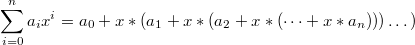double sin3(double x)
{
double x2 = x * x;
return x * (a0 + x2 * (a1 + x2 * (a2 + x2 * (a3 + x2 * (a4 + x2 * (a5 + x2 * (a6 + x2 * a7)))))));
}


We are down to 9 multiplications and 7 additions. There is probably no way to be faster, is there? Let’s see…

## Timings

Here are the timings in nanoseconds for the above code, compared with the glibc’s sin() function. The test CPU is an Intel® Core™ i7-2620M CPU at 2.70GHz. The functions were compiled using -O3 -ffast-math:

 function sin sin1 sin2 sin3 nanoseconds per call 22.518 16.406 16.658 25.276

Wait, what? Our superbly elegant function, performing only 9 multiplications, is actually slower than the 64-multiplication version? Which itself is as fast as the 16-multiplication one? Surely we overlooked something.

That’s right. We ignored the CPU pipeline.

## The instruction pipeline

In order to execute an instruction, such as “add A and B into C”, a CPU needs to do at least the following:

• fetch the instruction (ie. read it from the program’s memory)
• decode the instruction
• read the instruction’s operands (ie. A and B)
• execute the instruction
• write the result in memory or in registers (in our case, C)

On a modern Intel® CPU, the execution step only accounts for 1/10 or even 1/16 of the total execution time. The idea behind pipelining is simple: while executing an instruction, the CPU can often already read the operands for the next one.

But there is a problem with this strategy: if the next instruction depends on the result of the current one, the CPU cannot read the next operands yet. This is called a read-after-write hazard, and most usually causes a pipeline stall: the CPU just does nothing until it can carry on.

For the sake of simplicity, imagine the CPU’s pipeline depth is 3. At a given time, it can fetch, execute and finish one instruction:

 ● instruction is being fetched, executed or finished ○ instruction could start, but needs to wait for the result of a previous instruction

This is how the CPU would execute A = (a + b) * (c + d):

 time → total: 7 1 B = a + b ● ● ● 2 C = c + d ● ● ● 3 A = B * C ○ ○ ● ● ●

The c + d operation can be started very early because it does not depend on the result of a + b. This is called instruction-level parallelism. However, the final B * C operation needs to wait for all previous instructions to finish.

Since every operation in sin3() depends on the previous one, this is how it would execute that function:

 time → total: 48 1 x2 = x * x ● ● ● 2 A = a7 * x2 ○ ○ ● ● ● 3 A += a6 ○ ○ ● ● ● 4 A *= x2 ○ ○ ● ● ● 5 A += a5 ○ ○ ● ● ● 6 A *= x2 ○ ○ ● ● ● 7 A += a4 ○ ○ ● ● ● 8 A *= x2 ○ ○ ● ● ● 9 A += a3 ○ ○ ● ● ● 10 A *= x2 ○ ○ ● ● ● 11 A += a2 ○ ○ ● ● ● 12 A *= x2 ○ ○ ● ● ● 13 A += a1 ○ ○ ● ● ● 14 A *= x2 ○ ○ ● ● ● 15 A += a0 ○ ○ ● ● ● 16 A *= x ○ ○ ● ● ●

These 9 multiplications and 7 additions are done in 48 units of time. No instruction-level parallelism is possible because each instruction needs to wait for the previous one to finish.

The secret behind sin2()’s performance is that the large number of independent operations allows the compiler to reorganise the computation so that the instructions can be scheduled in a much more efficient way. This is roughly how GCC compiled it:

 time → total: 30 1 x2 = x * x ● ● ● 2 A = a7 * x2 ○ ○ ● ● ● 3 x3 = x2 * x ● ● ● 4 A += a6 ○ ● ● ● 5 B = a1 * x3 ● ● ● 6 x5 = x3 * x2 ● ● ● 7 A *= x2 ● ● ● 8 C = a2 * x5 ○ ● ● ● 9 B += x ● ● ● 10 x7 = x5 * x2 ● ● ● 11 A += a5 ● ● ● 12 D = a3 * x7 ● ● ● 13 B += C ● ● ● 14 x9 = x7 * x2 ● ● ● 15 B += D ○ ● ● ● 16 E = a4 * x9 ● ● ● 17 x11 = x9 * x2 ● ● ● 18 B += E ○ ● ● ● 19 A *= x11 ● ● ● 20 A += B ○ ○ ● ● ●

These 13 multiplications and 7 additions are executed in 30 units of time instead of 48 for the previous version. The compiler has been rather clever here: the number of ○’s is kept small.

Note that 30 / 48 = 0.625, and the ratio between sin2 and sin3’s timings is 16.658 / 25.276 = 0.659. Reality matches theory pretty well!

## Going further

We have seen that increasing the number of operations in order to break dependencies between CPU instructions allowed to help the compiler perform better optimisations taking advantage of the CPU pipeline. But that was at the cost of 40% more multiplications. Maybe there is a way to improve the scheduling without adding so many instructions?

Luckily there are other ways to evaluate a polynomial.

## Even-Odd form and similar schemes

Consider our 8th order polynomial: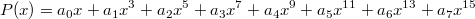Separating the odd and even coefficients, it can be rewritten as: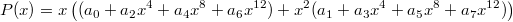Which using Horner’s form yields to: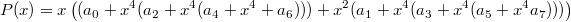This polynomial evaluation scheme is called the Even-Odd scheme. It only has 9 multiplications and 7 additions (only one multiplication more than the optimal case). It results in the following C code:

double sin4(double x)
{
double x2 = x * x;
double x4 = x2 * x2;
double A = a0 + x4 * (a2 + x4 * (a4 + x4 * a6));
double B = a1 + x4 * (a3 + x4 * (a5 + x4 * a7));
return x * (A + x2 * B);
}


And this is the expected scheduling:

 time → total: 33 1 x2 = x * x ● ● ● 2 x4 = x2 * x2 ○ ○ ● ● ● 3 B = a7 * x4 ○ ○ ● ● ● 4 A = a6 * x4 ● ● ● 5 B += a5 ○ ● ● ● 6 A += a4 ● ● ● 7 B *= x4 ○ ● ● ● 8 A *= x4 ● ● ● 9 B += a3 ○ ● ● ● 10 A += a2 ● ● ● 11 B *= x4 ○ ● ● ● 12 A *= x4 ● ● ● 13 B += a1 ○ ● ● ● 14 A += a0 ● ● ● 15 B *= x2 ○ ● ● ● 16 A += B ○ ○ ● ● ● 17 A *= x ○ ○ ● ● ●

Still not good enough, but we’re certainly onto something here. Let’s try another decomposition for the polynomial: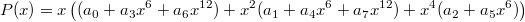And using Horner’s form again: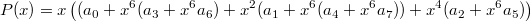Resulting in the following code:

double sin5(double x)
{
double x2 = x * x;
double x4 = x2 * x2;
double x6 = x4 * x2;
double A = a0 + x6 * (a3 + x6 * a6);
double B = a1 + x6 * (a4 + x6 * a7);
double C = a2 + x6 * a5;
return x * (A + x2 * B + x4 * C);
}


And the following scheduling:

 time → total: 31 1 x2 = x * x ● ● ● 2 x4 = x2 * x2 ○ ○ ● ● ● 3 x6 = x4 * x2 ○ ○ ● ● ● 4 B = x6 * a7 ○ ○ ● ● ● 5 A = x6 * a6 ● ● ● 6 C = x6 * a5 ● ● ● 7 B += a4 ● ● ● 8 A += a3 ● ● ● 9 C += a2 ● ● ● 10 B *= x6 ● ● ● 11 A *= x6 ● ● ● 12 C *= x4 ● ● ● 13 B += a1 ● ● ● 14 A += a0 ● ● ● 15 B *= x2 ○ ● ● ● 16 A += C ● ● ● 17 A += B ○ ○ ● ● ● 18 A *= x ○ ○ ● ● ●

One more instruction and two units of time better. That’s slightly better, but still not as good as we would like. One problem is that a lot of time is lost waiting for the value x6 to be ready. We need to find computations to do in the meantime to avoid pipeline stalls.

## High-Low form

Instead of splitting the polynomial into its even and odd coefficients, we split it into its high and low coefficients:And again using Horner’s form:The corresponding code is now:

double sin6(double x)
{
double x2 = x * x;
double x4 = x2 * x2;
double x8 = x4 * x4;
double A = a0 + x2 * (a1 + x2 * (a2 + x2 * a3));
double B = a4 + x2 * (a5 + x2 * (a6 + x2 * a7));
return x * (A + x8 * B);
}


And the expected scheduling:

 time → total: 30 1 x2 = x * x ● ● ● 2 B = x2 * a7 ○ ○ ● ● ● 3 A = x2 * a3 ● ● ● 4 x4 = x2 * x2 ● ● ● 5 B += a6 ● ● ● 6 A += a2 ● ● ● 7 x8 = x4 * x4 ● ● ● 8 B *= x2 ● ● ● 9 A *= x2 ● ● ● 10 B += a5 ○ ● ● ● 11 A += a1 ● ● ● 12 B *= x2 ○ ● ● ● 13 A *= x2 ● ● ● 14 B += a4 ○ ● ● ● 15 A += a0 ● ● ● 16 B *= x8 ○ ● ● ● 17 A += B ○ ○ ● ● ● 18 A *= x ○ ○ ● ● ●

Finally! We now schedule as well as GCC, and with 11 multiplications instead of 13. Still no real performance gain, though.

## Pushing the limits

Can we do better? Probably. Remember that each ○ in the above table is a pipeline stall, and any instruction we would insert there would be basically free.

Note the last instruction, A *= x. It causes a stall because it needs to wait for the final value of A, but it would not be necessary if A and B had been multiplied by x beforehands.

Here is a way to do it (bold instructions indicate a new instruction or a modified one):

 time → total: 27 1 x2 = x * x ● ● ● 2 B = x2 * a7 ○ ○ ● ● ● 3 A = x2 * a3 ● ● ● 4 x4 = x2 * x2 ● ● ● 5 B += a6 ● ● ● 6 A += a2 ● ● ● 7 x8 = x4 * x4 ● ● ● 8 B *= x2 ● ● ● 9 A *= x2 ● ● ● 10 x3 = x2 * x ● ● ● 11 B += a5 ● ● ● 12 A += a1 ● ● ● 13 C = a0 * x ● ● ● 14 B *= x2 ● ● ● 15 A *= x3 ● ● ● 16 x9 = x8 * x ● ● ● 17 B += a4 ● ● ● 18 A += C ● ● ● 19 B *= x9 ○ ● ● ● 20 A += B ○ ○ ● ● ●

Excellent! Just as many instructions as GCC, but now with fewer pipeline stalls. I don’t know whether this scheduling is optimal for the (incorrect) assumption of a 3-stage pipeline, but it does look pretty good. Also, loading a0, a1 etc. from memory hasn't been covered for the sake of simplicity.

Anyway, we just need to write the code corresponding to this behaviour, and hope the compiler understands what we need:

double sin7(double x)
{
double x2 = x * x;
double x3 = x2 * x;
double x4 = x2 * x2;
double x8 = x4 * x4;
double x9 = x8 * x;
double A = x3 * (a1 + x2 * (a2 + x2 * a3));
double B = a4 + x2 * (a5 + x2 * (a6 + x2 * a7));
double C = a0 * x;
return A + C + x9 * B;
}


## Conclusion

It’s time to check the results! Here they are, for all the functions covered in this article:

 function sin sin1 sin2 sin3 sin4 sin5 sin6 sin7 nanoseconds per call 22.518 16.406 16.658 25.276 18.666 18.582 16.366 17.470

Damn. All these efforts to understand and refactor a function, and our best effort actually performs amongst the worst!

What did we miss? Actually, this time, nothing. The problem is that GCC didn't understand what we were trying to say in sin7() and proceeded with its own optimisation ideas. Compiling with -O3 instead of -O3 -ffast-math gives a totally different set of timings:

 function sin sin1 sin2 sin3 sin4 sin5 sin6 sin7 nanoseconds per call 22.497 30.250 19.865 25.279 18.587 18.958 16.362 15.891

There. We win eventually!

There is a way to still use -ffast-math yet prevent GCC from trying to be too clever. This might be preferable because we do not want to lose the benefits of -ffast-math in other places. By using an architecture-specific assembly construct, we can mark temporary variables as used, effectively telling GCC that the variable needs to be really computed and not optimised away:

double sin7(double x)
{
double x2 = x * x;
double x3 = x2 * x;
double x4 = x2 * x2;
double x8 = x4 * x4;
double x9 = x8 * x;
#if defined __x86_64__
__asm__("" : "+x" (x3), "+x" (x9));
#elif defined __powerpc__ || defined __powerpc64__
__asm__("" : "+f" (x3), "+f" (x9));
#else
__asm__("" : "+m" (x3), "+m" (x9)); /* Out of luck :-( */
#endif
double A = x3 * (a1 + x2 * (a2 + x2 * a3));
double B = a4 + x2 * (a5 + x2 * (a6 + x2 * a7));
double C = a0 * x;
return A + C + x9 * B;
}


This works on the x86_64 architecture, where "+x" indicates the SSE registers commonly used for floating point calculations, and on the PowerPC, where "+f" can be used. This approach is not portable and it is not clear what should be used on other platforms. Using "+m" is generic but often means a useless store into memory; however, on x86 it is still a noticeable gain.

And our final results, this time with the full -O3 -ffast-math optimisation flags:

 function sin sin1 sin2 sin3 sin4 sin5 sin6 sin7 nanoseconds per call 22.522 16.411 16.663 25.277 18.628 18.588 16.365 15.617